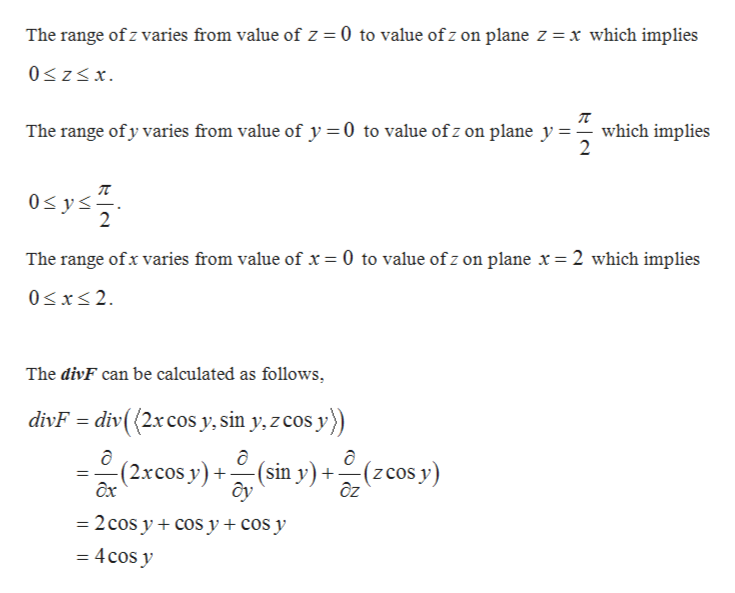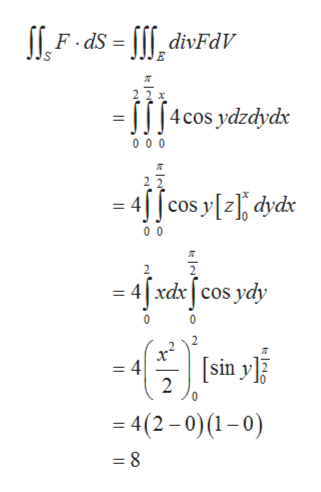Decide which integral of the Divergence Theorem to use and compute the outward flux of the vector fieldF=<2xcosy, siny, zcosy>across the surface​S, where S is the boundary of the region bounded by the planesx=2​, y=​0, y=π​/2, z=​0, and z=x

Question

Decide which integral of the Divergence Theorem to use and compute the outward flux of the vector field

F=<2xcosy, siny, zcosy>

across the surface​S, where S is the boundary of the region bounded by the planes

x=2​, y=​0, y=π​/2, z=​0, and z=x
Step 1

From the Divergence theorem, for a positively oriented boundary surface (outward Normal) of region E the flux is equal to triple integral of divF over the region E.

Step 2

Find the range of x,y and z as follows.help_outlineImage TranscriptioncloseThe range of z varies from value of z 0 to value ofz on plane z = x which implies 0zx The range of y varies from value of y 0 to value of z on plane y which implies 2 0s ys 2 The range ofx varies from value of x = 0 to value ofz on plane x = 2 which implies 0xs2 The divF can be calculated as follows divF = div( (2xcos y, sin y, z cos y)) (sin y) +(zcos y) ôy (2.xcos y) x ôz =2 cos y cos y + cos y = 4cos y fullscreen
Step 3

Outward flux can be calcu...help_outlineImage TranscriptioncloseFds- divFdV =I1[4cos ydzdydx 0 0 0 2 2 4[f cos y[, dvdx 0 0 4 xdxcos ydy x = 4 2 sin y 0 = 4(2-0)(1-0) = 8 fullscreen

Want to see the full answer?

See Solution

Want to see this answer and more?

Our solutions are written by experts, many with advanced degrees, and available 24/7

See Solution
Tagged in

Calculus